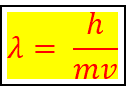# Problem: An alpha particle (mass = 6.6 x 10 −24 g) emitted by a radium isotope travels at 3.4 x 107 ± 0.1 x 10 7 mi/h. What is its de Broglie wavelength (in meters)?

###### FREE Expert Solution

The de Broglie equation is an equation used to describe the wave properties of matter. The de Broglie equation is as follows:Where λ is the wavelength of the particle (m),

is the Plank’s constant (6.626 x 10-34 mkg / s)

m is the mass of the particle (kg)

is the speed of the particle (m/s)

79% (99 ratings)###### Problem Details

An alpha particle (mass = 6.6 x 10 −24 g) emitted by a radium isotope travels at 3.4 x 107 ± 0.1 x 10 7 mi/h. What is its de Broglie wavelength (in meters)?

What scientific concept do you need to know in order to solve this problem?

Our tutors have indicated that to solve this problem you will need to apply the De Broglie Wavelength concept. You can view video lessons to learn De Broglie Wavelength. Or if you need more De Broglie Wavelength practice, you can also practice De Broglie Wavelength practice problems.

What is the difficulty of this problem?

Our tutors rated the difficulty ofAn alpha particle (mass = 6.6 x 10 −24 g) emitted by a radiu...as medium difficulty.

What professor is this problem relevant for?

Based on our data, we think this problem is relevant for Professor Hill's class at MSCD.

What textbook is this problem found in?

Our data indicates that this problem or a close variation was asked in Chemistry: The Molecular Nature of Matter and Change - Silberberg 8th Edition. You can also practice Chemistry: The Molecular Nature of Matter and Change - Silberberg 8th Edition practice problems.